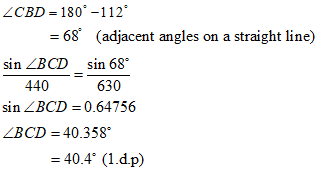top of page
Search

# 3 O-Level Secondary Math Topics to Focus On

Updated: Feb 21It’s no secret that preparing for O-Level Math exams is stressful. The sheer amount of information your child needs to absorb in Secondary school can be overwhelming. There is so much your child needs to ‘focus’ on that finding a starting point can be challenging.

For this blog post, we sought advice from one of our most experienced O-Level Math tuition teachers, Mr Jackson Toh from AGrader Jurong West. While he recognises that different students would struggle with a range of topics, he’s shared three situations where students often make mistakes during their exams. This usually arises from not fully understanding different mathematical concepts or getting misled by exam questions.

### 1. Proving Similar and Congruent TrianglesWhen approaching a question on the topic of proving similar or congruent triangles, one of the most critical mistakes students make is to develop assumptions on certain geometrical information based on how they appear on the diagram provided. This includes stating that certain lines are parallel, or certain angles are equal without providing any geometrical reasons for it. An example of this is shown with the following exam question:

The diagram provided below is a rhombus ABCD. The question requires the students to prove and explain why triangle ADE and triangle CDE are congruent (meaning they are of the same shape and size).Looking at the diagram, it is very easy for students to state that the lines AE and EC are of equal length without providing any geometrical reasons because the lines appear so. This will cost them the question in the exam!

The truth is, the lines AE and AC are actually the “trap” to mislead the students. The correct solution to the question, as shown below, does not even require the lines AE and EC.At AGrader, our worksheets are designed to support students in preparing for their exams and feature questions similar to those they’re likely to face. With practice, our students get more exposure to such exam questions and gain a better understanding of the maths concepts. Our educators also constantly remind and educate them to build strong fundamentals in their understanding of the topic.

With more understanding, students are better prepared to tackle similar questions in their exams and avoid repeating the same mistakes.

### 2. Mastering Trigonometry and its ApplicationsThere are many Geometry questions in O-Level Math that require students to find certain unknowns of a triangle. This includes topics such as Bearings, Elevation and also Radian measures involving finding triangles or segments of a circle.

In addition to these topics, it’s especially important for students to build strong foundations in applying the Sine Rule and Cosine Rule in Trigonometry to find unknown angles or sides of a triangle.

As diagrams start to look increasingly complex, trying to work out an unknown in a triangle can get more confusing. But there is a simple trick to it!

Every triangle has three interior angles and three sides.

At AGrader, students learn to go through the checklist below to see if the following information has been provided about triangles in the question:

1. One Side and Two Interior Angles OR

2. Two Sides and One Interior Angle OR

3. Three Sides Given

If so, students would then be able to use either Sine Rule or/and Cosine Rule to determine the unknowns in the question. An example of this application is shown below:In the diagram above, students are asked to find the angle BCD. As observed, the triangle BCD only has two known sides BD=440m and CD=630m given. Here’s how our educators would guide students through the question with the aid of the checklist above:

1. We have two known sides given in the triangle BCD.

2. From the checklist case (b), we know that finding another angle in the triangle BCD will allow us to apply the Sine Rule to find the angle BCD.

3. Can we find another angle in the triangle BCD?

4. Yes! Angle CBD can be found due to adjacent angles on a straight line.

5. Now we can use the Sine Rule to find angle BCD!When learning a concept in school, students may not fully comprehend how to apply certain formulas that certain questions require.

By using a simple checklist with our educators’ guidance, students are able to understand the concept more easily. With strong fundamentals, they’re more likely to apply the formula accurately.

### 3. Finding the Area of a Regular PolygonWhen preparing for exams, students in Singapore may encounter questions in Secondary Math that require them to comprehend and apply multiple concepts. These concepts are often taught separately during their Secondary Math journey, causing difficulties for students. With the help of secondary maths tuition in Singapore, students can gain the necessary skills and knowledge to tackle these types of questions with ease.

A good example is the concept of “Finding the Area of a Regular Polygon” where students need to apply their knowledge of Polygons they learned in Secondary 1, and technical skills to find unknown angles and sides using Trigonometry, which is learned in Secondary 3. An example of such a question is shown below:At AGrader Learning Centre, students get to practise a variety of tricky exam questions that are not commonly found in textbooks. With our educators’ guidance, students get exposure to worked solutions – just like you’ve seen in this blog post. This prepares them to apply different concepts even if these concepts aren’t commonly used together.

We offer students worked solutions to help scaffold the learning in O-level Math tuition classes. This stage is part of the tuition centre’s unique learner-directed curriculum that is developed in accordance with the latest MOE syllabus. Find out more about how our classes can help your child improve their O-Level Math here.